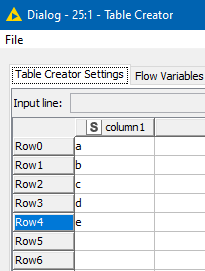# unable to get value from cell in dataframe

Hi KNIMErs’

I am unable to get a value from cell by referring to the row number and column name in KNIME python script node. However, when I refer to the cell using column number and row number, it works fine. This is a bit weird.
When I use .loc or .at function, eg input_table_1.at[1,“column1”], it gives key error.
However, .iloc or .iat works fine. eg. input_table_1.iloc[1,0]

Thanks

Deepan

Hi @AJA, and welcome to the community!

Would you be able to upload a small example workflow with some data (it can be just using a table creator and just a few rows and columns of dummy data) along with a small python script exhibiting the issue you’ve described, as that’ll make it easier to assist.

If you don’t know how to do that… you need to export the workflow in Knime, and then use the upload button here on the forum to upload the exported. knwf file. Please ask if you need further assistance with that.

Thanks.

2 Likes

Hi @AJA,

I’ve looked at this further, and the “key error” that you are getting is expected from the “at” function on a dataframe if a label that you have supplied is not known.

The reason you are seeing this is because of the value `1` in your `.at[1,"column1"]` statement

Ordinarily, when using pandas, when you create a dataframe from a list, or csv file, for example, the “index” for the dataframe will simply be an integer which effectively acts as a row number which I believe is what you were expecting.

However, in KNIME, it creates an index column containing the “row label” which is not an integer, but is instead a label “Row0”, “Row1”. This can then act as a unique row identifier regardless of future actions such as sorting, joining and filtering.

To demonstrate what I mean, take the following following input table:In a python script, if you were to print input_table_1 in the Python Script node, you would see it looks like this:

This means that the first parameter for the at function will be one of the row labels “Row0”, “Row1” and so on, whilst the second parameter would be the column label “column1”
e.g.

If you want to return the cell for a named column, using a row number (starting at row 0), the following methods could be used

``````# Copy input to output
output_table_1 = input_table_1.copy()

print(input_table_1)

print("-- returning specific cell value:")
print("Using at:"+input_table_1.at["Row1","column1"])

# To return a given cell by row number and column name, you can use one
# of the following methods:

print("Using iLoc#1:"+input_table_1.iloc[1,input_table_1.columns.get_loc("column1")])
print("Using iLoc#2:"+input_table_1['column1'].iloc)

``````

I’ve uploaded the above example here:
python cell value in dataframe.knwf (8.0 KB)

edit: if you really want to use “at”, then the following would also work:

`print("Using at and row number:"+input_table_1.at[input_table_1.index,'column1'])`

5 Likes

Hi Takbb,
Thanks loads for helping me understand row and column labels of KNIME tables!!! Solution suggested by you works perfectly fine!

Regards
AJA

2 Likes

This topic was automatically closed 7 days after the last reply. New replies are no longer allowed.